## Example Questions

← Previous 1 3

### Example Question #1 : How To Find The Volume Of A Cube

A cubic box has sides of length x. Another cubic box has sides of length 4x. How many of the boxes with length x could fit in one of the larger boxes with side length 4x?

64

40

4

16

80

64

Explanation:

The volume of a cubic box is given by (side length)3. Thus, the volume of the larger box is (4x)3 = 64x3, while the volume of the smaller box is x3. Divide the volume of the larger box by that of the smaller box, (64x3)/(x3) = 64.

### Example Question #2 : How To Find The Volume Of A Cube

I have a hollow cube with 3” sides suspended inside a larger cube of 9” sides.  If I fill the larger cube with water and the hollow cube remains empty yet suspended inside, what volume of water was used to fill the larger cube?

73 in3

702 in3

72 in3

698 in3

216 in3

702 in3

Explanation:

Determine the volume of both cubes and then subtract the smaller from the larger.  The large cube volume is 9” * 9” * 9” = 729 in3 and the small cube is 3” * 3” * 3” = 27 in3.  The difference is 702 in3.

### Example Question #3 : How To Find The Volume Of A Cube

A cube weighs 5 pounds. How much will a different cube of the same material weigh if the sides are 3 times as long?

10 pounds

15 pounds

45 pounds

135 pounds

135 pounds

Explanation:

A cube that has three times as long sides is 3x3x3=27 times bigger than the original. Therefore, the answer is 5x27= 135.

### Example Question #1 : How To Find The Volume Of A Cube

If the volume of a cube is 50 cubic feet, what is the volume when the sides double in length?

500 cu ft

100 cu ft

200 cu ft

400 cu ft

300 cu ft

400 cu ft

Explanation:

Using S as the side length in the original cube, the original is s*s*s. Doubling one side and tripling the other gives 2s*2s*2s for a new volume formula for 8s*s*s, showing that the new volume is 8x greater than the original.

### Example Question #5 : How To Find The Volume Of A Cube

A cube has 2 faces painted red and the remaining faces painted green. The total area of the green faces is 36 square inches. What is the volume of the cube in cubic inches?

54

16

27

8

64

27

Explanation:

Cubes have 6 faces. If 2 are red, then 4 must be green. We are told that the total area of the green faces is 36 square inches, so we divide the total area of the green faces by the number of green faces (which is 4) to get the area of each green face: 36/4 = 9 square inches. Since each of the 6 faces of a cube have the same size, we know that each edge of the cube is √9 = 3 inches. Therefore the volume of the cube is 3 in x 3 in x 3 in = 27 cubic inches.

### Example Question #6 : How To Find The Volume Of A Cube

If a waterproof box is 50cm in length, 20cm in depth, and 30cm in height, how much water will overflow if 35 liters of water are poured into the box?

15 liters

1 liters

30 lites

No water will flow out of the box

5 liters

5 liters

Explanation:

The volume of the box is 50 * 20 * 30 cm = 30,000 cm3.

1cm3 = 1mL, 30,000 cm3 = 30,000mL = 30 L.

Because the volume of the box is only 30 L, 5 L of the 35 L will not fit into the box.

### Example Question #7 : How To Find The Volume Of A Cube

Kim from Idaho can only stack bales of hay in her barn for 3 hours before she needs a break. She stacks the bales at a rate of 2 bales per minute, 3 bales high with 5 bales in a single row. How many full rows will she have at the end of her stacking?

27

16

20

15

24

24

Explanation:

She will stack 360 bales in 3 hours. One row requires 15 bales. 360 divided by 15 is 24.

### Example Question #8 : How To Find The Volume Of A Cube

A cube has a volume of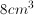. What is the volume of cube with sides that are twice as long?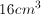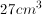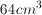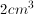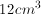Explanation:

The volume of a cube is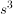.

If each side of the cube is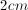, then the volume will be.

If we double each side, then each side would be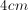and the volume would be.

### Example Question #9 : How To Find The Volume Of A Cube

How many  smaller boxes with a dimensions of  1 by 5 by 5 can fit into cube shaped box with a surface area of 150?

4

8

7

6

5

5

Explanation:

There surface are of a cube is 6 times the area of one face of the cube , therefore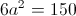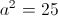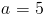a is equal to an edge of the cube

the volume of the cube is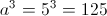The problem states that the dimensions of the smaller boxes are 1 x 5 x 5, the volume of one of the smaller boxes is 25.

Therefore, 125/25 = 5 small boxes

### Example Question #10 : How To Find The Volume Of A Cube

If a cube has its edges increased by a factor of 5, what is the ratio of the new volume to the old volume?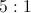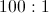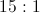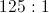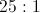A cubic volume is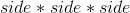. Let the original sides be 1, so that the original volume is 1. Then find the volume if the sides measure 5.  This new volume is 125.  Therefore, the ratio of new volume to old volume is 125: 1.